# NCERT Solutions for Class 9 Science Chapter 4 Structure Of The Atom

NCERT Solutions for Class 9 Science Chapter 4 Structure Of The Atom

Class 9 Science NCERT Textbook Page 47

Question:- 1. What are canal rays?

Answer:- Canal rays are positively charged radiation that lead to the discovery of the proton which a positively charged subatomic particle.

Question:- 2. If an atom contains one electron and one proton, will it carry any charge or not?

Answer:- Because a proton is positively charged and an electron is negatively charged, the net charge becomes neutral as the both the charged particles cancel each other out.

Class 9 Science NCERT Textbook Page 49

Question:- 1. On the basis of Thomson’s model of an atom, explain how the atom is neutral as a whole.

Answer:- According to Thomson’s atom model, an atom has both negative and positive charges of equal quantity and magnitude. As a result, they balance each other, resulting in an electrically neutral atom.

Question:- 2. On the basis of Rutherford’s model of an atom, which subatomic particle is present in the nucleus of an atom?

Answer:- The positively charged protons

Question:- 3. Draw a sketch of Bohr’s model of an atom with three shells.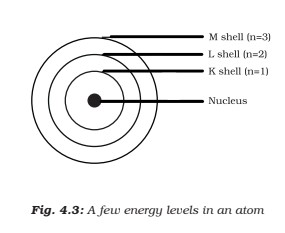Question:- 4. What do you think would be the observation if the α-particle scattering experiment is carried out using a foil of a metal other than gold?

Answer:- When any other metal foil is used instead of gold in the – particle scattering experiment, the results are the same. This is due to the fact that when an atom is examined separately, its structure remains the same.

Class 9 Science NCERT Textbook Page 49

Question:- 1. Name the three sub-atomic particles of an atom.

Answer:- The positively charged – Protons

The negatively charged – Electrons

The neutral in nature ( no charge ) – Neutrons

Question:- 2. Helium atom has an atomic mass of 4 u and two protons in its nucleus. How many neutrons does it have?

Answer:-  Atomic mass = number of protons + number of neutrons

4 = 2 + number of neutrons

4 – 2 = Number of neutrons

= 2 Hence, Helium has 2 neutrons.

Class 9 Science NCERT Textbook Page 50

Question:- 1. Write the distribution of electrons in carbon and sodium atoms.

Answer:- The total number of electrons in a carbon atom = 6

So, distribution of electrons in carbon atom is given by:

No. of electron in first orbit or K-shell = 2

No. of electron in second orbit or L-shell = 4

Hence the eletronic distribution of carbon atom is 2, 4.

The total number of electrons in a sodium atom = 11

So, distribution of electrons in sodium atom is given by:

No. of electron in first orbit or K-shell = 2

No. of electron in second orbit or L-shell = 8

No. of electron in third orbit or M-shell = 8

Hence the eletronic distribution of sodium atom is 2, 8, 1.

Question:- 2. If K and L shells of an atom are full, then what would be the total number of electrons in the atom?

Answer:- The maximum capacity of K shell = 2 electrons

and the maximum capacity of  L shell = 8 electrons

As a result, when both shells are full, the total number of electrons in the atom is 2+8 = 10.

Class 9 Science NCERT Textbook Page 52

Question:- 1. How will you find the valency of chlorine, sulphur and magnesium?Class 9 Science NCERT Textbook Page 52

Question:- 1. If number of electrons in an atom is 8 and number of protons is also 8, then (i) what is the atomic number of the atom? and (ii) what is the charge on the atom?

Answer:- (i) The atomic number = The number of protons.

Therefore, the atomic number of the atom is 8.

(ii) Since the number electrons = protons

therefore, the charge on the atom is 0.

Question:- 2. With the help of Table 4.1, find out the mass number of oxygen and sulphur atom.

Answer:- Mass number of oxygen = Number of protons + Number of neutrons

Mass number of oxygen = 8 + 8

Mass number of oxygen = 16

Mass number of sulphur = Number of protons + Number of neutrons

Mass number of sulphur = 16 +16

Mass number of sulphur = 32

Class 9 Science NCERT Textbook Page 53

Question:- 1. For the symbol H,D and T tabulate three sub-atomic particles found in each of them.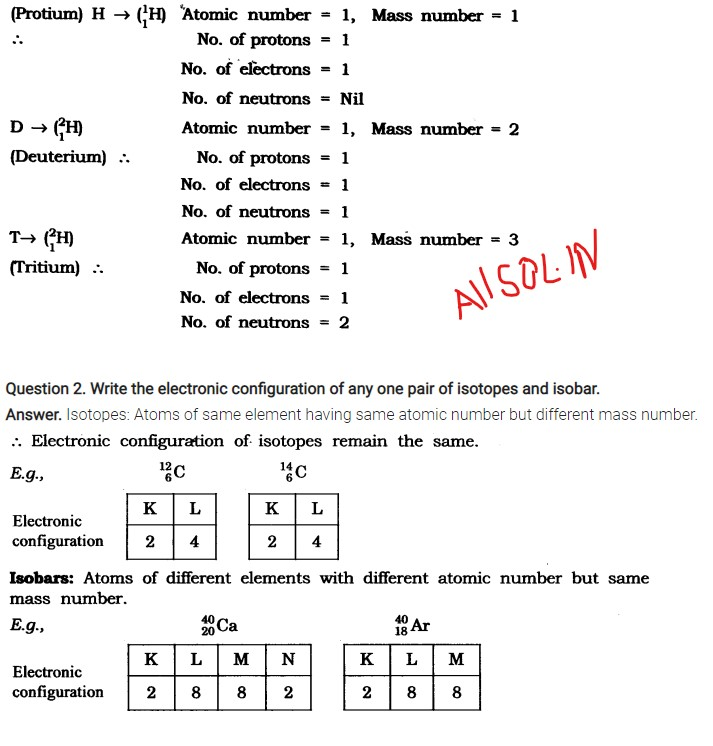Exercises

Question:- 1. Compare the properties of electrons, protons and neutrons.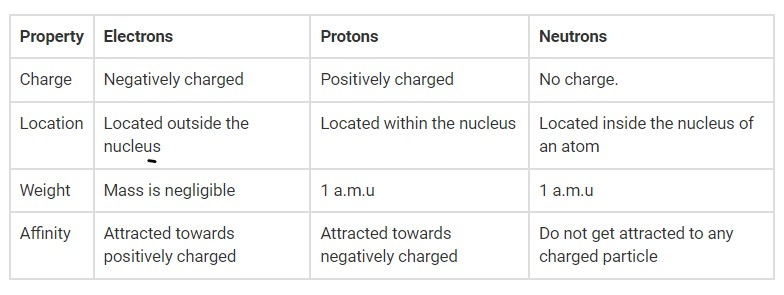Question:- 2. What are the limitations of J.J. Thomson’s model of the atom?

Answer:- According to J.J. Thomson’s model of an atom, the electrons are embedded all over in the positively charged spheres. However the experiments done by other scientists showed that protons are present only in the nuclues that is in centre of the atom and electrons are distributed around it.

Question:- 3. What are the limitations of Rutherford’s model of the atom?

Answer:- According to Rutherford’s model of an atom, the electrons of an atom revolve in a circular orbit around the nucleus. Any rotating particle would experience acceleration and release energy. The circling electron would eventually lose its energy and fall into the nucleus, making the atom exceedingly unstable. However, we know that atoms are extremely stable.

Question:- 4. Describe Bohr’s model of the atom.

Answer:-  Bohr’s model of the atom :-

1. The nucleus is in the centre of an atom.
2. The nucleus is surrounded by negatively charged electrons.
3. When electrons are in their orbits, they do not emit energy.
4. The distinct orbits are designated by the letters K, L, M, and N. n=1, 2, 3, 4 are the numbers used to represent them.
5. The maximum number of electrons present in a shell is given by the formula 2n2 , where ‘n’ is the orbit number or energy level index, 1,2,3,…. Hence the maximum number of electrons in different shells are as follows: first orbit or K-shell will be = 2 × 12 = 2, second orbit or L-shell will be = 2 × 22 = 8, third orbit or M-shell will be = 2 × 32 = 18, fourth orbit or N-shell will be = 2 × 42 = 32, and so on.
6. The maximum number of electrons that can be accommodated in the outermost orbit is 8.
7. Electrons are not accommodated in a given shell, unless the inner shells are filled. That is, the shells are filled in a step-wise manner

Question:- 5. Compare all the proposed models of an atom given in this chapter.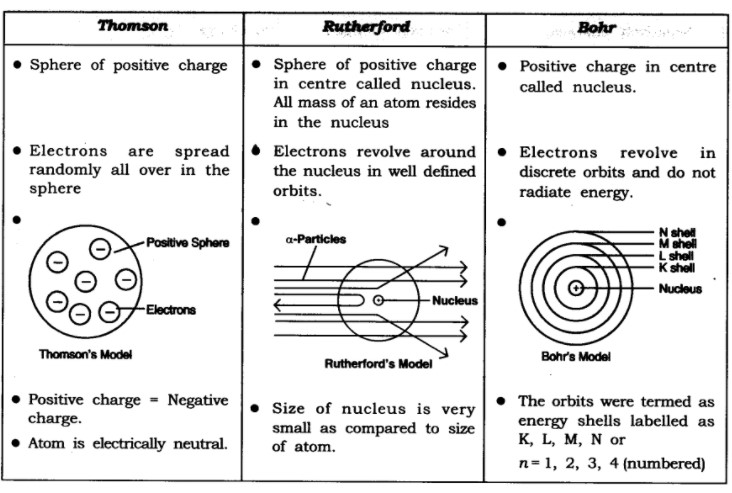Question:- 6. Summarise the rules for writing of distribution of electrons in various shells for the first eighteen elements.

Answer:- The rules for writing of distribution of electrons in various shells for the first eighteen elements are following:-

1. The maximum number of electrons present in a shell is given by the formula 2n2 , where ‘n’ is the orbit number or energy level index, 1,2,3,…. Hence the maximum number of electrons in different shells are as follows: first orbit or K-shell will be = 2 × 12 = 2, second orbit or L-shell will be = 2 × 22 = 8, third orbit or M-shell will be = 2 × 32 = 18, fourth orbit or N-shell will be = 2 × 42 = 32, and so on.
2. The maximum number of electrons that can be accommodated in the outermost orbit is 8.
3. Electrons are not accommodated in a given shell, unless the inner shells are filled. That is, the shells are filled in a step-wise manner

Question:- 7. Define valency by taking examples of silicon and oxygen.

Answer:- The combining capacity of the element is called the valency.

Silicon’s electrical configuration is 2,8,4.

As a result, silicon has a valency of 4 because these electrons can be shared with others to complete an octet.

Oxygen valency: It has an electrical configuration of 2,6.

As a result, oxygen has a valency of two since it will gain two electrons to complete its octet.

Question:- 8. Explain with examples (i) Atomic number, (ii) Mass number, (iii) Isotopes and (iv) Isobars. Give any two uses of isotopes.

Answer:- (i)  Atomic number :- The atomic number is defined as the total number of protons present in the nucleus of an atom. It is denoted by letter “Z”.  For example:- Oxygen has 8 protons in its nucleus, so its atomic number is 8.

(ii) Mass number :- The mass number is defined as the sum of the total number of protons and neutrons present in the nucleus of an atom. It is denoted by letter ‘A’. For example, mass of carbon is 12 because it has 6 protons and 6 neutrons, 6 u + 6 u = 12 u.

(iii) Isotopes :- Elements which have the same atomic number but different mass numbers are called Isotopes. For example:- protium (11H), deuterium (12H or D) and tritium (13H or T).

(iv) Isobars :- Isobars are atoms which have the same mass number but different atomic numbers. For example:- 2040Ca and  1840Ar.

Two uses of Isotopes are following:-

• In nuclear reactors, an isotope of uranium is used as a fuel.
• In the treatment of cancer, a cobalt isotope is used.
• In the treatment of goitre, an isotope of iodine is used.

(write any two)

Question:- 9. Na+ has completely filled K and L shells. Explain.

Answer:- Atomic number of Na =11

Its electronic configuration = K L M – 2 8 1

Sodium atom (Na) has to loose 1 electron to become stable and form Na+ ion. Hence it has completely filled K and L shells.

Question:- 10. If bromine atom is available in the form of, say, two isotopes 7935 Br (49.7%) and 8135 Br (50.3%), calculate the average atomic mass of bromine atom.

Answer:- Average atomic mass of the bromine atom =  (79 × 49.7)/100 + (81 × 50.3)/100

= 39.26 u+ 40.64u

=79.9u

Question:- 11. The average atomic mass of a sample of an element X is 16.2 u. What are the percentages of isotopes 168 X and 188 X in the sample?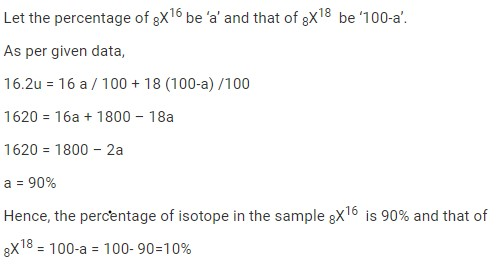Question:- 12. If Z = 3, what would be the valency of the element? Also, name the element.

Answer:- Z (atomic number) = 3,

Then electronic configuration = 2, 1

Valency = 1

Name of the element is lithium.

Question:- 13. Composition of the nuclei of two atomic species X and Y are given as under

X            Y

Protons =     6              6

Neutrons =   6              8

Give the mass numbers of X and Y. What is the relation between the two species?

Answer:- Mass number of X = Protons + Neutrons = 6 + 6 = 12

Mass number of Y = Protons + Neutrons = 6 + 8 = 14

As the atomic number of both element is same i.e., = 6.

Then both X and Y are isotopes of same element.

Question:- 14. For the following statements, write T for True and F for False.

(a) J.J. Thomson proposed that the nucleus of an atom contains only nucleons.

(b) A neutron is formed by an electron and a proton combining together. Therefore, it is neutral.

(c) The mass of an electron is about 1/2000 times that of proton.

(d) An isotope of iodine is used for making tincture iodine, which is used as a medicine.

Put tick  against correct choice and cross against wrong choice in questions 15, 16 and 17

1. False
2. False
3. True
4. False

Question:- 15. Rutherford’s alpha-particle scattering experiment was responsible for the discovery of

(a) Atomic Nucleus     (b) Electron

(c) Proton                     (d) Neutron

Answer:- (a) Atomic Nucleus

Question:- 16. Isotopes of an element have

(a) the same physical properties

(b) different chemical properties

(c) different number of neutrons

(d) different atomic numbers.

Answer:- (c) different number of neutrons

Question:- 17. Number of valence electrons in Cl ion are:

(a) 16    (b) 8    (c) 17    (d) 18

Question:- 18. Which one of the following is a correct electronic configuration of sodium?

(a) 2,8    (b) 8,2,1    (c) 2,1,8    (d) 2,8,1.